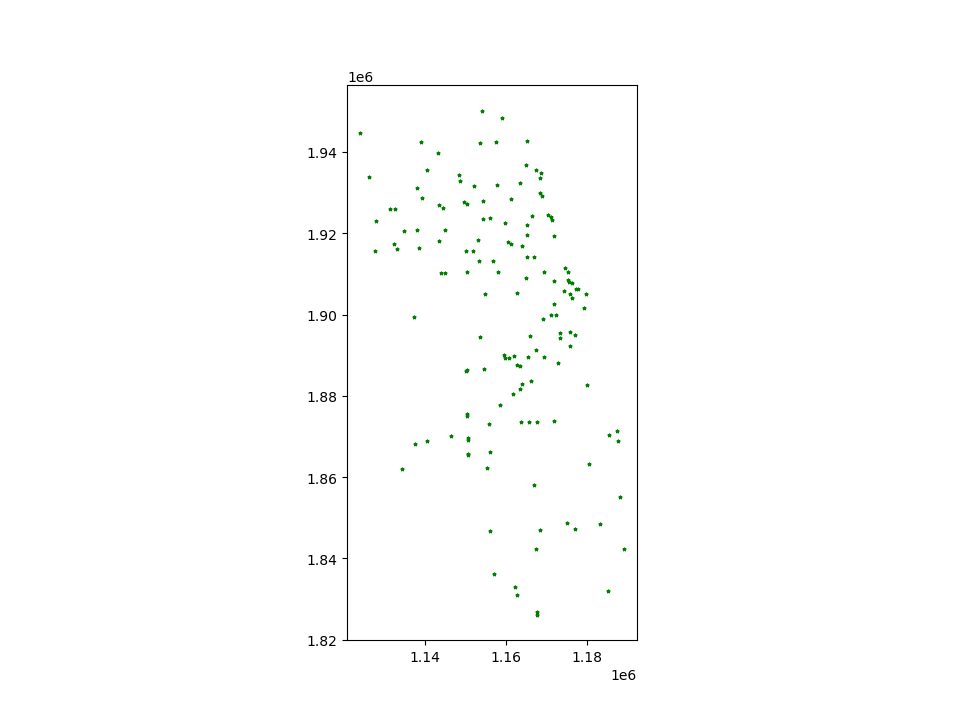# Mapping Tools¶

geopandas provides a high-level interface to the `matplotlib` library for making maps. Mapping shapes is as easy as using the `plot()` method on a `GeoSeries` or `GeoDataFrame`.

```In : world = geopandas.read_file(geopandas.datasets.get_path('naturalearth_lowres'))

In : cities = geopandas.read_file(geopandas.datasets.get_path('naturalearth_cities'))
```

We can now plot those GeoDataFrames:

```# Examine country GeoDataFrame
Out:
pop_est                        ...                                                                   geometry
0  28400000.0                        ...                          POLYGON ((61.21081709172574 35.65007233330923,...
1  12799293.0                        ...                          (POLYGON ((16.32652835456705 -5.87747039146621...
2   3639453.0                        ...                          POLYGON ((20.59024743010491 41.85540416113361,...
3   4798491.0                        ...                          POLYGON ((51.57951867046327 24.24549713795111,...
4  40913584.0                        ...                          (POLYGON ((-65.50000000000003 -55.199999999999...

[5 rows x 6 columns]

# Basic plot, random colors
In : world.plot();
```Note that in general, any options one can pass to pyplot in `matplotlib` (or style options that work for lines) can be passed to the `plot()` method.

## Choropleth Maps¶

geopandas makes it easy to create Choropleth maps (maps where the color of each shape is based on the value of an associated variable). Simply use the plot command with the `column` argument set to the column whose values you want used to assign colors.

```# Plot by GDP per capta
In : world = world[(world.pop_est>0) & (world.name!="Antarctica")]

In : world['gdp_per_cap'] = world.gdp_md_est / world.pop_est

In : world.plot(column='gdp_per_cap');
```### Choosing colors¶

One can also modify the colors used by `plot` with the `cmap` option (for a full list of colormaps, see the matplotlib website):

```In : world.plot(column='gdp_per_cap', cmap='OrRd');
```The way color maps are scaled can also be manipulated with the `scheme` option (if you have `pysal` installed, which can be accomplished via `conda install pysal`). The `scheme` option can be set to ‘equal_interval’, ‘quantiles’ or ‘percentiles’. See the PySAL documentation for further details about these map classification schemes.

```In : world.plot(column='gdp_per_cap', cmap='OrRd', scheme='quantiles');
```## Maps with Layers¶

There are two strategies for making a map with multiple layers – one more succinct, and one that is a little more flexible.

Before combining maps, however, remember to always ensure they share a common CRS (so they will align).

```# Look at capitals
# Note use of standard `pyplot` line style options
In : cities.plot(marker='*', color='green', markersize=5);

# Check crs
In : cities = cities.to_crs(world.crs)

# Now we can overlay over country outlines
# And yes, there are lots of island capitals
# apparently in the middle of the ocean!
```Method 1

```In : base = world.plot(color='white', edgecolor='black')

In : cities.plot(ax=base, marker='o', color='red', markersize=5);
```Method 2: Using matplotlib objects

```In : import matplotlib.pyplot as plt

In : fig, ax = plt.subplots()

# set aspect to equal. This is done automatically
# when using *geopandas* plot on it's own, but not when
# working with pyplot directly.
In : ax.set_aspect('equal')

In : world.plot(ax=ax, color='white', edgecolor='black')
Out: <matplotlib.axes._subplots.AxesSubplot at 0x7fb7ac190048>

In : cities.plot(ax=ax, marker='o', color='red', markersize=5)
Out: <matplotlib.axes._subplots.AxesSubplot at 0x7fb7ac190048>

In : plt.show();
```## Other Resources¶

Links to jupyter Notebooks for different mapping tasks:

Making Heat Maps